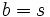# All transpositions involving one element generate the finitary symmetric group

Jump to: navigation, search

## Statement

Suppose$S$ is a non-empty set,$s \in S$ an element, and$G = \operatorname{FSym}(S)$ is the Finitary symmetric group (?) on$S$. Then, the set of all Transposition (?)s in$S$ involving the element$s$ is a generating set for$G$.

When$S$ is finite, this generating set is both a Sims-reduced generating set and a Jerrum-reduced generating set.

## Facts used

1. Transpositions generate the finitary symmetric group

## Proof

Given: A non-empty set$S$, an element$s \in S$.$G$ is the finitary symmetric group on$S$.$T$ is the subset of$G$ comprising all transpositions involving$S$.

To prove:$T$ generates$G$.

Proof:

1. Every transposition is in the subgroup generated by$T$: Consider a transposition$(a,b)$, with$a,b \in S$. If either$a = s$ or$b = s$,$(a,b) \in T$. If neither equals$s$, we still have$(a,b) = (s,a)(s,b)(s,a)$, with all elements on the right side being in$T$. Thus,$(a,b)$ is in the subgroup generated by$T$.
2. The previous step and fact (1) yield that the subgroup generated by$T$ is the whole group$G$.